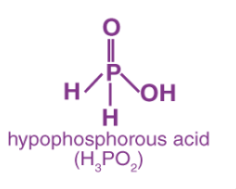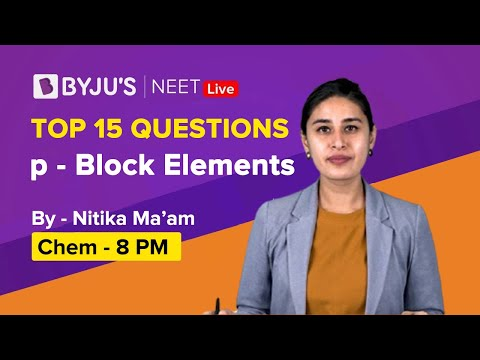Jet Set Go! All about Aeroplanes Jet Set Go! All about Aeroplanes

# P Block Elements Questions

The elements present in the periodic table from group 13 to group 18 are called P block elements. The valence electrons of these elements enter the outermost p subshell and thus are called P block elements. Each group of the P block family is known by unique names. The Group 13 elements (Boron family) are called Icosagens, Group 14 elements (Carbon family) are called Crystallogens, Group 15 elements (Nitrogen family) are called Pnictogens, Group 16 elements (Oxygen family) are called Chalcogens, Group 17 elements are called Halogens and Group 18 elements are the famous noble gases. Each group of the P block has its own properties and various uses.

 Definition: The group 13 consists of elements B, Al, Ga, In Tl, Nh. The group 14 consists of elements C, Si, Ge, Sn, Pb, Fl. The group 15 consists of elements N, P, As, Sb, Bi, Mc. The group 16 elements consists of O, S, Se, Te,Po, Lv. The group 17 consist of elements F, Cl, Br, I, At, Ts. The group 18 elements consist of He, Ne, Ar,Kr, Xe, Rn, Og.

## P Block Elements Chemistry Questions with Solutions

Q1. Why do noble gases have low boiling points?

Answer: Noble gases are mono-atomic gases. They do not have intermolecular forces and have very weak London dispersion forces. Thus, they have low boiling points.

Q2. Why is white phosphorus more reactive than red phosphorus?

Answer: White phosphorus is more reactive than red phosphorus due to the presence of angle strain in it. The angle is 60o instead of the 109.5o seen in standard tetrahedral geometry. Red Phosphorus forms a chain-like polymeric network of tetrahedral units bonded covalently and thus is more stable and less reactive than white phosphorus.

Q3. Determine the oxidation number of Phosphorus in H3PO2 molecule.

Answer: The oxidation number of phosphorus in H3PO2 is calculated as shown below:

Oxidation number of Hydrogen is: 1

Oxidation number of Oxygen is: -2

Let Oxidation number of phosphorus be x.

1(3) + x + 2(-2) = 0

3 + x – 4 = 0

x = +1.

Therefore, the oxidation number of phosphorus is +1 in H3PO2.

Q4. What is the structure of the XeF2 molecule?

Answer: The structure of the XeF2 molecule is given below.

Q5. Explain the reducing behavior of H3PO2 by a reaction.

Answer: H3PO2 reduces silver nitrate to elemental silver as shown in the reaction below.

$$\begin{array}{l}4AgNO_{3} + 2H_{2}O + H_{3}PO_{2}\rightarrow 4Ag + 4HNO_{3} + H_{3}PO_{4}\end{array}$$

Greater the number of P-H bonds, the greater the reducing power of oxyacids of Phosphorus. H3PO2 has 2 P-H bonds, thus it acts as a strong reducing agent and itself gets oxidized to +5 oxidation state.Q6. Identify the gas released on heating Pb(NO3)2.

Answer: A brown colored NO2 gas is released when lead nitrate is heated.

$$\begin{array}{l}2Pb(NO_{3})_{2} \overset{\Delta }{\rightarrow} 2PbO +4NO_{2} + O_{2}\end{array}$$

Q7. Choose the correct statement

1. HCl is a weaker acid than HF
2. On going down group 17, boiling point increases
3. Cl has lower electron affinity than F
4. Cl2 has lower dissociation energy than F2

Reason: On going down the group, the size of halogens increases. Due to increase in size, van der Waals forces of attraction also increase, leading to an increase in boiling points.

Q8. Which molecule given below does not produce nitrogen on heating?

1. (NH4)Cr2O7
2. NH4Cl + NaNO2
3. NH4Cl + CaO
4. Ba(N3)2

The reaction of NH4Cl + CaO is given below:

NH4Cl +CaO → H2O + NH3 +CaCl2

Q9. Which chemical depletes the ozone layer?

1. Sulfur
2. Hydrogen
3. Nitrogen
4. Chlorofluorocarbons (CFCs)

CFCs are harmful chemicals responsible for warming the lower layer of the atmosphere causing global warming and they also deplete the ozone layer which protects the earth from harmful UV-rays of the sun.

Q10. Which catalyst is used in the contact process for the production of sulfuric acid?

1. V2O5
2. Al2O3
3. SO3
4. Fe2O3

Answer: (a) V2O5 increases the rate of the reaction and also reduces the need for high temperatures.

Q11. Why is phosphorus relatively reactive compared to nitrogen?

Answer: Nitrogen is less reactive than Phosphorus because nitrogen has a triple bond and phosphorus has a single bond. It requires a higher amount of energy to break the bonds of nitrogen and thus they are chemically inert.

Q12. Match the following items of column 1 with column 2 and choose the correct answer:

Column 1

Column 2

1. Sulfur

A) Tear gas

1. Sulphuric acid

B) Storage batteries

1. Chlorine gas

C) Chalcogen

1. Trichloronitromethane

D) Highest electron gain enthalpy

Column 1

Column 2

1. Sulfur

C) Chalcogen

1. Sulphuric acid

B) Storage batteries

1. Chlorine gas

D) Highest electron gain enthalpy

1. Trichloronitromethane

A) Tear gas

Q13. Match the following items of column 1 with column 2 and choose the correct answer:

Column 1 (Molecule)

Column 2 (Bond angle in degrees)

1. NH3

a) 109.5

1. H2O

b) 180

1. CH4

c) 104.5

1. CO2

d) 107

Column 1 (Molecule)

Column 2 (Bond angle in degrees)

1) NH3

a) 107

2) H2O

b) 104.5

3) CH4

c) 109.5

4) CO2

d) 180

Q14. Write the preparation methods of XeO3 and XeOF4

Synthesis of XeO3: XeO3 can be synthesized by 2 ways-

$$\begin{array}{l}6XeF_{4} + 12H_{2}O \to 4Xe + 2XeO_{3} + 24HF + 3O_{2}\end{array}$$
$$\begin{array}{l}XeF_{6} + 3H_{2}O \to XeO_{3} + 6HF\end{array}$$

Synthesis of XeOF4:

$$\begin{array}{l}XeF_{6} + H_{2}O \to XeOF_{4} + 2HF\end{array}$$

Q15. Explain the industrial preparation of Ammonia.

Ammonia is produced in large quantities industrially using a process called Haber’s process. In this process, atmospheric nitrogen is combined with hydrogen reversibly to form ammonia. Iron is used as a catalyst and molybdenum is used as a promoter for this reaction. It is carried out under high pressure (200 atm – 400 atm) and high temperature (500o C). It is a highly efficient process and gives 98% conversion efficiency. The reaction is given below:

$$\begin{array}{l}N_{2} + 3H_{2} \rightleftharpoons 2NH_{3}\end{array}$$

## Practise Questions on P block elements

Q1. What are group 15 elements collectively called?

1. Chalcogens
2. Pnictogens
3. Halogens
4. Icosagens

Q2. What is the trend in ionic radii for As(3+), Bi(3+) and Sb(3+)

Q3. What are the relative acidic strengths of HCl, HBr, HF and HI?

Q4. What is the chemical formula of oleum?

1. H2SO5
2. H3S2O6
3. H2S2O7
4. H2S2O8

Q5. Write the electronic configuration of oxygen, nitrogen and sulphur.

Click the PDF to check the answers for Practice Questions.

## P Block Elements NEET Questions